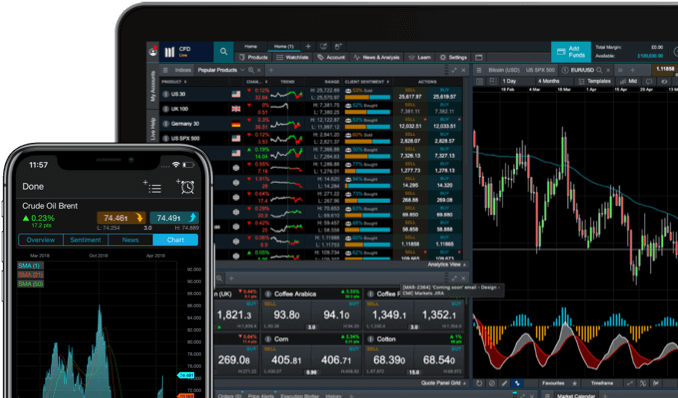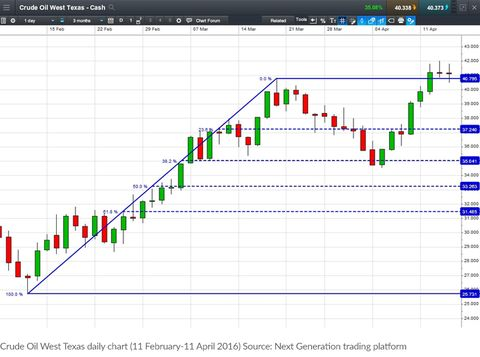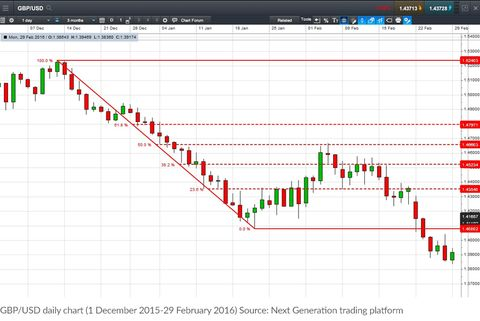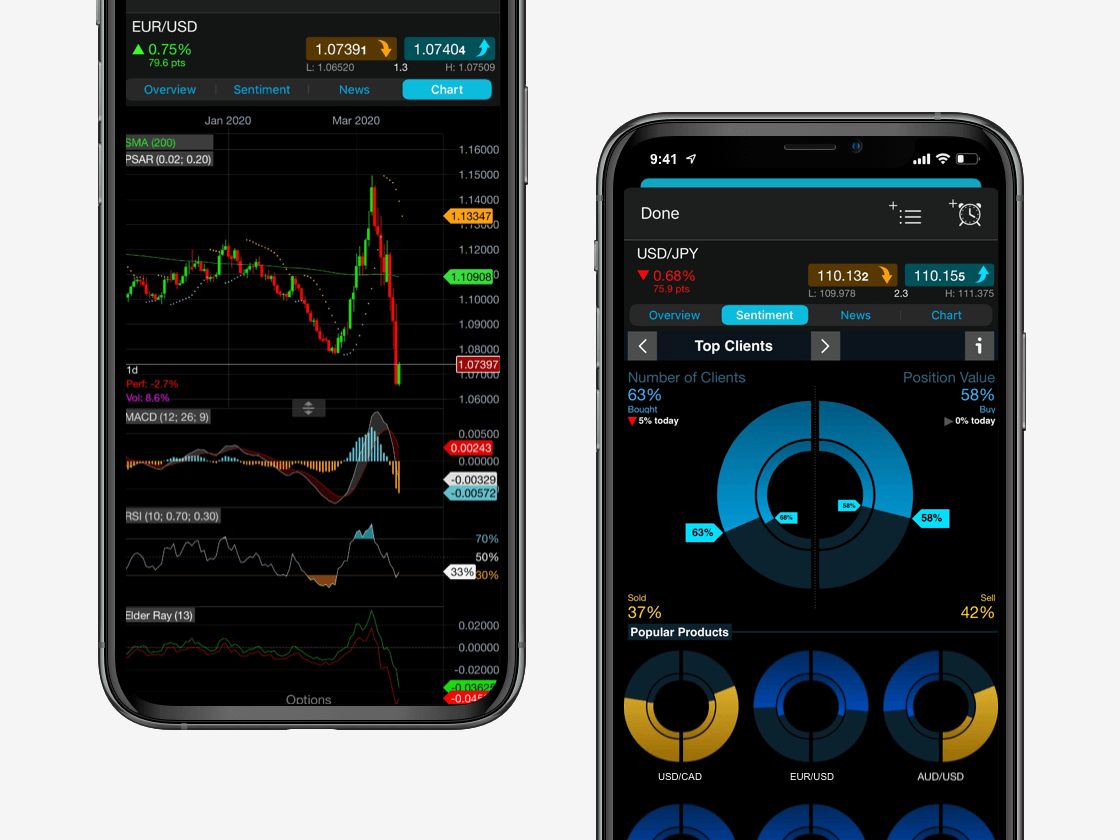Spread bets and CFDs are complex instruments and come with a high risk of losing money rapidly due to leverage. 73% of retail investor accounts lose money when spread betting and/or trading CFDs with this provider. You should consider whether you understand how spread bets and CFDs work and whether you can afford to take the high risk of losing your money.

# How to trade with Fibonacci

Fibonacci retracements are a popular form of technical analysis used by traders in order to predict future potential prices in the financial markets. If used correctly, Fibonacci retracements and ratios can help traders to identify upcoming support and resistance levels based on past price action.

It’s important to remember that Fibonacci lines are a confirmation tool. For this reason, the indicator is best used alongside other technical analysis tools such as trend lines, volume, moving average convergence divergence (MACD) and moving averages. Generally speaking, the greater the number of confirming indicators, the stronger the trade signal is likely to be.

See inside our platform

Get tight spreads, no hidden fees and access to 9,300+ instruments.## What is Fibonacci in trading?

Leonardo Fibonacci was a mathematician born in 1170 AD. From his work, we get the Fibonacci sequence of numbers, and also the well-known Fibonacci golden ratio. The Fibonacci sequence is a series of numbers where the next number is simply the sum of the two preceding numbers. So for example, it would run 0, 1, 1, 2, 3, 5, 8, 13, 21, 34, 55, 89, 144 and so on, with the sequence continuing indefinitely.

It is based on the rate of reproduction of two theoretical rabbits and the subsequent population growth if the following generations continued to reproduce. At first glance, it may seem somewhat confusing to find that there is a connection between a 12th century mathematician, the rate at which rabbits reproduce and predicting the future direction of the financial markets using technical analysis. So, why is this series of numbers so important for traders?## Fibonacci's golden ratio

The focus tends to be on the ratio between the numbers in the sequence. This is considered to be the most important part of Fibonacci's work. Any number in the series divided by the previous number gives us 1.618 as we get further down the series. This is known as the 'Fibonacci golden ratio'. For Fibonacci followers, there are plenty of examples in nature adhering to this ratio (or the inverse of the number, 0.618). It seems to have played an enormous part in the building blocks of everything around us.​

For example, if you divide the number of female bees by male bees in a hive, you will get 1.618 as the answer. For sunflowers, each new seed is 0.618 of a turn from the last one. Fibonacci also applies to humans as well. There are lots of instances of this golden ratio working in relation to our bodies: one example is the ratio of the length of your forearm to your hand, which is 1.618.

### Fibonacci’s golden ratio example

In financial markets, the Fibonacci golden ratio has the same mathematical base as the natural phenomena mentioned above. When traders use the golden ratio in their technical analysis, the ratio is usually translated into three percentages: 38.2% (often rounded to 38%), 50% and 61.8% (usually rounded to 62%). Having said that, traders can use more multiples when necessary, such as: 23.6%, 161.8%, 423%, 684.4% and so on.

The 38.2% ratio is found by dividing one number in the series by the number two places to the right. For example, 21 divided by 55 equals 0.382. The 23.6% ratio divides one number in the series by the number three places to the right. For example, 8 divided by 34 equals 0.235.

## Fibonacci retracement levels

The argument of Fibonacci followers is: if so much of nature and the world is made up of these Fibonacci ratios, surely the same would apply to the markets too? Analysts can use this approach when learning to trade Fibonacci through its retracements. Let's say for example that a market has risen and, similar to all markets, it doesn't move in a straight line and starts to fall back. Traders will look at Fibonacci ratios to try and figure out where the fall may stop and the market will resume its previous rise.

Fibonacci retracement levels often mark retracement reversal points with surprising accuracy. The retracement levels are a powerful tool that can be applied to all timeframes, including day trading and long-term investing. Fibonacci numbers also play a crucial role in the Elliott Wave principle, a technical analysis tool used to identify market cycles. The tool can be used across many different asset classes, such as foreign exchange, shares, commodities and indices.

Join a trading community committed to your success

## What is the Fibonacci sequence?

The golden ratio of 1.618 – the magic number – gets translated into three percentages: 23.6%, 38.2% and 61.8%. These are the three most popular percentages, although some traders will also look at the 50% and 76.4% levels. 50% is not a Fibonacci number, but has proved to be a generally popular number when correcting a primary or secondary price move. For now, we will focus on the 50% and the two more popular Fibonacci percentages of 38.2% and 61.8%.

These are then applied to the chart to try and figure out potential hidden levels of support or resistance in the market. When the market drops back to 38.2% of its previous rise (the first major Fibonacci retracement), traders will check to see if any buyers come in. If this 38.2% level gets broken, then the expectation is for the 50% retracement to be the next target. If the market slides through that 50% retracement level, then traders will look to see if the market finally stops its decline when it has retraced 61.8% of the prior move. For most Fibonacci followers, if it breaks through that 61.8% level, it means that the market direction is going back to where it started.

We can create Fibonacci retracements by taking a peak and trough (or two extreme points) on a chart and dividing the vertical distance by the above key Fibonacci ratios. Once these trading patterns are identified, horizontal lines can be drawn and then used to identify possible support and resistance levels.

### What is the Fibonacci sequence used for?

The Fibonacci sequence and golden ratio appear frequently in nature, biology, architecture and fine art. It is seen in flower petals, tree branches, human DNA and population growth. The golden ratio and other Fibonacci ratios are also often found in the financial markets, and they form the foundation of the Fibonacci retracement tool.

## How to use Fibonacci retracements in trading

• Fibonacci retracement lines can be created when you divide the vertical distance between the high and low points by the key Fibonacci ratios. Horizontal lines are drawn on the trading chart​ at the 23.6%, 38.2% and 61.8% retracement levels. Some traders also like to use the 50.0% ratio. This is not really a Fibonacci ratio, but it can be useful. Often a security will retrace by around 50% before continuing its original trend.
• Charting software has simplified the process of drawing Fibonacci lines. Many trading platforms enable traders to plot Fibonacci lines. In an upward trend, you can select the Fibonacci line tool, select the low price and drag the cursor up to the high price. The indicator will mark key ratios such as 61.8%, 50.0% and 38.2% on the chart.
• Similarly, in a downward trend, you can select the Fibonacci line tool, choose the high price and drag the cursor down to the low price. The indicator will mark key ratios on the chart. To improve accuracy, traders can also use double tops or double bottoms as the high and low points.

### Fibonacci support and resistance

Fibonacci levels are mainly used to identify support and resistance levels. When a security is trending up or down, it usually pulls back slightly before continuing the trend. Often, it will retrace to a key Fibonacci retracement level such as 38.2% or 61.8%. These levels provide signals for traders to enter new positions in the direction of the original trend. In an uptrend, you might go long (buy) on a retracement down to a key support level. In a downtrend, you could look to go short (sell) when a security retraces up to its key resistance level. The tool works best when a security is trending up or down.

## Examples of the Fibonacci pattern

This example shows the rise in the price of Crude Oil West Texas (also called WTI Crude Oil), which is part of the commodities market​. The market then stalls, making it possible for traders to apply some Fibonacci retracements to that rally, to see where support comes in. As can be seen, the price does slide back but although briefly probing through, the 38.2% retracement in the \$35 area does end up providing some support. The market rebounds and moves out to fresh highs for the recovery.Fibonacci trading doesn't just apply to rising markets. If a market has fallen, then Fibonacci fans will apply the retracements to bounce back up. Let’s take an example of a market that has dropped 100 points. If it rallies 38.2%, then those looking at Fibonacci retracements will expect the rally to run out of steam. If that level is broken, then the 50% level is where traders would look for the market to turn back down. And finally, if that one gets broken then a 61.8% retracement of the down move is the next target, with a break here suggesting that the market will go all the way back to where it started the fall.

The next chart shows the drop in GBP/USD. The currency pair fell from the 1.5200 area to 1.4100 before stabilising. As the market stabilised, Fibonacci retracements could be applied to this fall. It can be seen that when the market had bounced back by 50%, the recovery ran out of steam and the slide resumed. This is an example of a Fibonacci retracement helping us to figure out when to sell short in a downtrend.## Best Fibonacci trading strategies

Fibonacci retracement lines are often used as part of trend-trading strategies. If a retracement is taking place within a trend, you could use the Fibonacci levels to place a trade in the direction of the underlying trend. The idea is that there is a higher chance a security’s price will bounce from the Fibonacci level back in the direction of the initial trend.

Fibonacci levels can be useful if a trader wants to buy a particular security but has missed out on a recent uptrend. In this situation, you could wait for a pullback. By plotting Fibonacci ratios such as 61.8%, 38.2% and 23.6% on a chart, traders may identify possible retracement levels and enter potential trading positions.

Fibonacci levels can be used across many different trading strategies, such as the following:

• Combining Fibonacci retracement lines with the MACD indicator. This strategy looks for a crossing over of the MACD indicator, when a security’s price touches an important Fibonacci level. When this happens, a position can be opened in the direction of the trend.
• Combining Fibonacci levels with the stochastic indicator. This two-line indicator can help identify overbought and oversold levels. The strategy looks for key signals from the stochastic indicator when the price touches an important Fibonacci level. The two signals together indicate an opportunity to open a position.
• Fibonacci retracement levels can be used across multiple timeframes, but are considered to be most accurate across longer timeframes. For example, a 38% retracement on a weekly chart is a more important technical level than a 38% retracement on a five-minute chart. Read more about choosing the right chart timeframes.

As with all technical analysis tools, Fibonacci retracement levels are most effective when used within a broader strategy. Using a combination of several indicators offers a chance to more accurately identify market trends, increasing the potential for profit. As a general rule, the more confirming factors, the stronger the trade signal.

Open an account to get started with Fibonacci trading.

## Summary

Not everyone is a fan of the Fibonacci approach to market analysis. Some just see the levels as a self-fulfilling prophecy as so many people are watching them, and not having any particular 'magical' properties. However, even for the sceptic, it can give an extra level of insight to potential market turning points that may not be clear at first glance. You should always consider risk management​ strategies when using technical indicators in trading.Powerful trading on the go

Seamlessly open and close trades, track your progress and set up alerts

CMC Markets is an execution-only service provider. The material (whether or not it states any opinions) is for general information purposes only, and does not take into account your personal circumstances or objectives. Nothing in this material is (or should be considered to be) financial, investment or other advice on which reliance should be placed. No opinion given in the material constitutes a recommendation by CMC Markets or the author that any particular investment, security, transaction or investment strategy is suitable for any specific person. The material has not been prepared in accordance with legal requirements designed to promote the independence of investment research. Although we are not specifically prevented from dealing before providing this material, we do not seek to take advantage of the material prior to its dissemination.

Join over 90,000 other committed traders

1

Apply for a live account

Complete our straightforward application form and verify your account

2

Fund your account

Deposit easily via debit card, bank transfer or PayPal

3

Find and trade

One touch, instant trading available on 9,300+ instruments